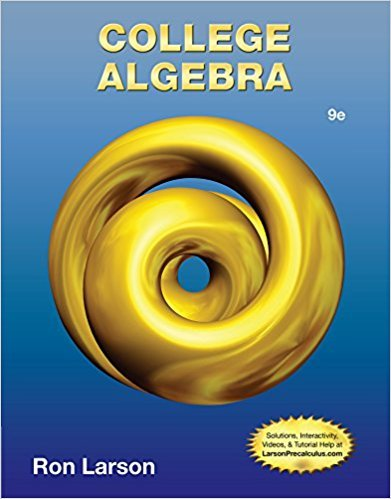×

×

Textbooks / Math / College Algebra 9

# College Algebra 9th Edition - Solutions by Chapter## Full solutions for College Algebra | 9th Edition

ISBN: 9781133963028College Algebra | 9th Edition - Solutions by Chapter

Solutions by Chapter
4 5 0 287 Reviews
##### ISBN: 9781133963028

The full step-by-step solution to problem in College Algebra were answered by , our top Math solution expert on 01/02/18, 09:21PM. This expansive textbook survival guide covers the following chapters: 9. Since problems from 9 chapters in College Algebra have been answered, more than 97433 students have viewed full step-by-step answer. This textbook survival guide was created for the textbook: College Algebra, edition: 9. College Algebra was written by and is associated to the ISBN: 9781133963028.

Key Math Terms and definitions covered in this textbook
• Back substitution.

Upper triangular systems are solved in reverse order Xn to Xl.

• Cofactor Cij.

Remove row i and column j; multiply the determinant by (-I)i + j •

• Distributive Law

A(B + C) = AB + AC. Add then multiply, or mUltiply then add.

• Fast Fourier Transform (FFT).

A factorization of the Fourier matrix Fn into e = log2 n matrices Si times a permutation. Each Si needs only nl2 multiplications, so Fnx and Fn-1c can be computed with ne/2 multiplications. Revolutionary.

• Fibonacci numbers

0,1,1,2,3,5, ... satisfy Fn = Fn-l + Fn- 2 = (A7 -A~)I()q -A2). Growth rate Al = (1 + .J5) 12 is the largest eigenvalue of the Fibonacci matrix [ } A].

• Gauss-Jordan method.

Invert A by row operations on [A I] to reach [I A-I].

• Gram-Schmidt orthogonalization A = QR.

Independent columns in A, orthonormal columns in Q. Each column q j of Q is a combination of the first j columns of A (and conversely, so R is upper triangular). Convention: diag(R) > o.

• Hessenberg matrix H.

Triangular matrix with one extra nonzero adjacent diagonal.

• Linear transformation T.

Each vector V in the input space transforms to T (v) in the output space, and linearity requires T(cv + dw) = c T(v) + d T(w). Examples: Matrix multiplication A v, differentiation and integration in function space.

• Normal equation AT Ax = ATb.

Gives the least squares solution to Ax = b if A has full rank n (independent columns). The equation says that (columns of A)·(b - Ax) = o.

• Nullspace N (A)

= All solutions to Ax = O. Dimension n - r = (# columns) - rank.

• Pivot columns of A.

Columns that contain pivots after row reduction. These are not combinations of earlier columns. The pivot columns are a basis for the column space.

• Positive definite matrix A.

Symmetric matrix with positive eigenvalues and positive pivots. Definition: x T Ax > 0 unless x = O. Then A = LDLT with diag(D» O.

• Rank r (A)

= number of pivots = dimension of column space = dimension of row space.

• Solvable system Ax = b.

The right side b is in the column space of A.

• Special solutions to As = O.

One free variable is Si = 1, other free variables = o.

• Spectrum of A = the set of eigenvalues {A I, ... , An}.

Spectral radius = max of IAi I.

• Subspace S of V.

Any vector space inside V, including V and Z = {zero vector only}.

• Triangle inequality II u + v II < II u II + II v II.

For matrix norms II A + B II < II A II + II B II·

• Vector space V.

Set of vectors such that all combinations cv + d w remain within V. Eight required rules are given in Section 3.1 for scalars c, d and vectors v, w.